What does it do?

Selects a specific value from the list of values provided

Formula breakdown:

=CHOOSE(index_num, value1, [value2], …)

What it means:

=CHOOSE(position to take from the list, first value, [second value and so on], …)

Suppose you have a list, and you want to dynamically retrieve values from your list based on the position, the CHOOSE formula in Excel is perfect for this!

It is very easy to use, and let me show you how to use this.

I explain how you can do this below:

STEP 1: We need to enter the CHOOSE function in a blank cell:

=CHOOSE(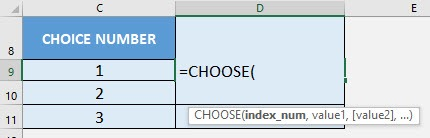STEP 2: The CHOOSE arguments:

index_num

What is the position to take from the list?

Select the cell containing the choice number. The maximum index you can put in is 254:

=CHOOSE(C9,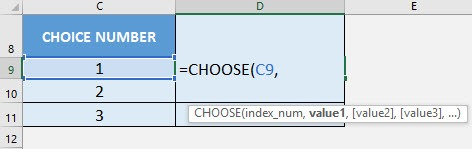value1, [value2], …

What are the values of the list?

In our example, let us type in our list that contains names of fruits:

=CHOOSE(C9, “apple”, “orange”, “grapes”, “lemon”)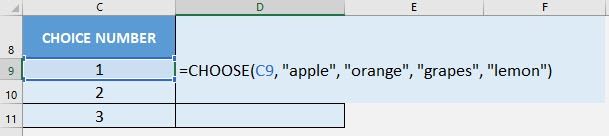Apply the same formula to the rest of the cells by dragging the lower right corner downwards.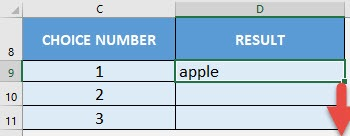We have now chosen the first, second and third items in our list!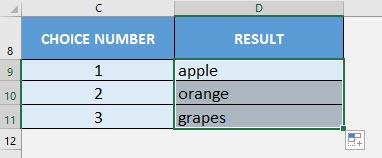How to Use the CHOOSE Formula in Excel